Equations reduced to pair of linear equations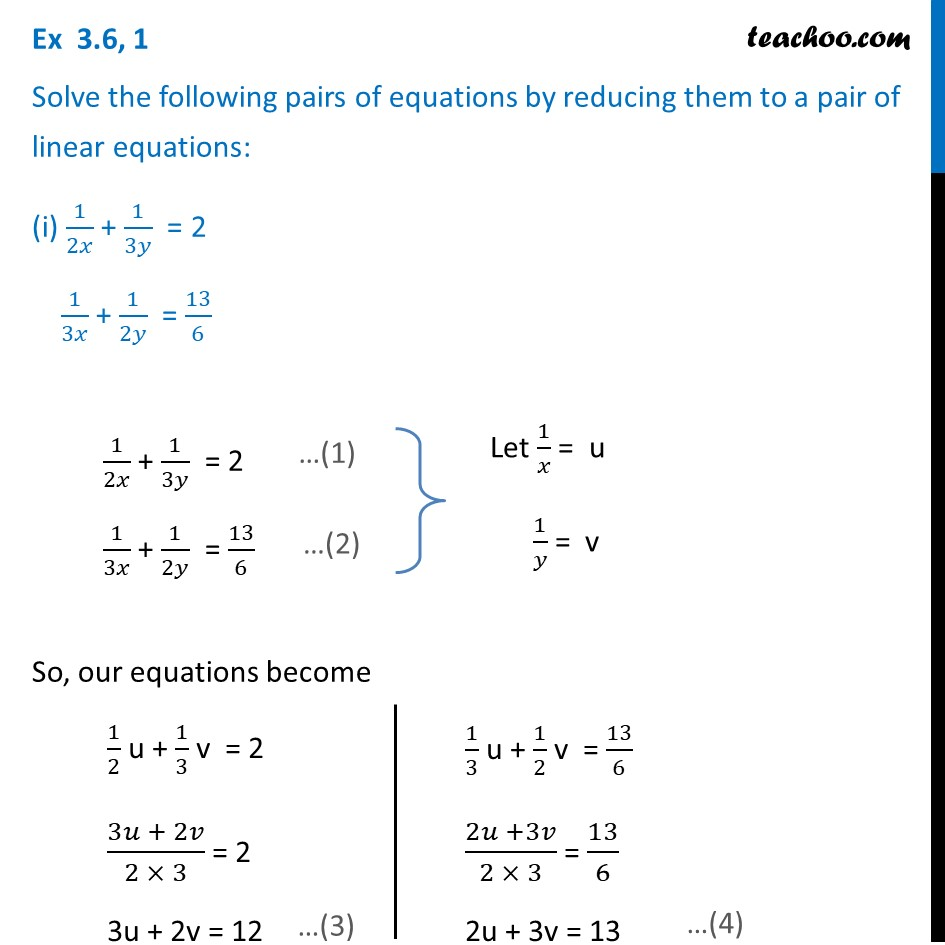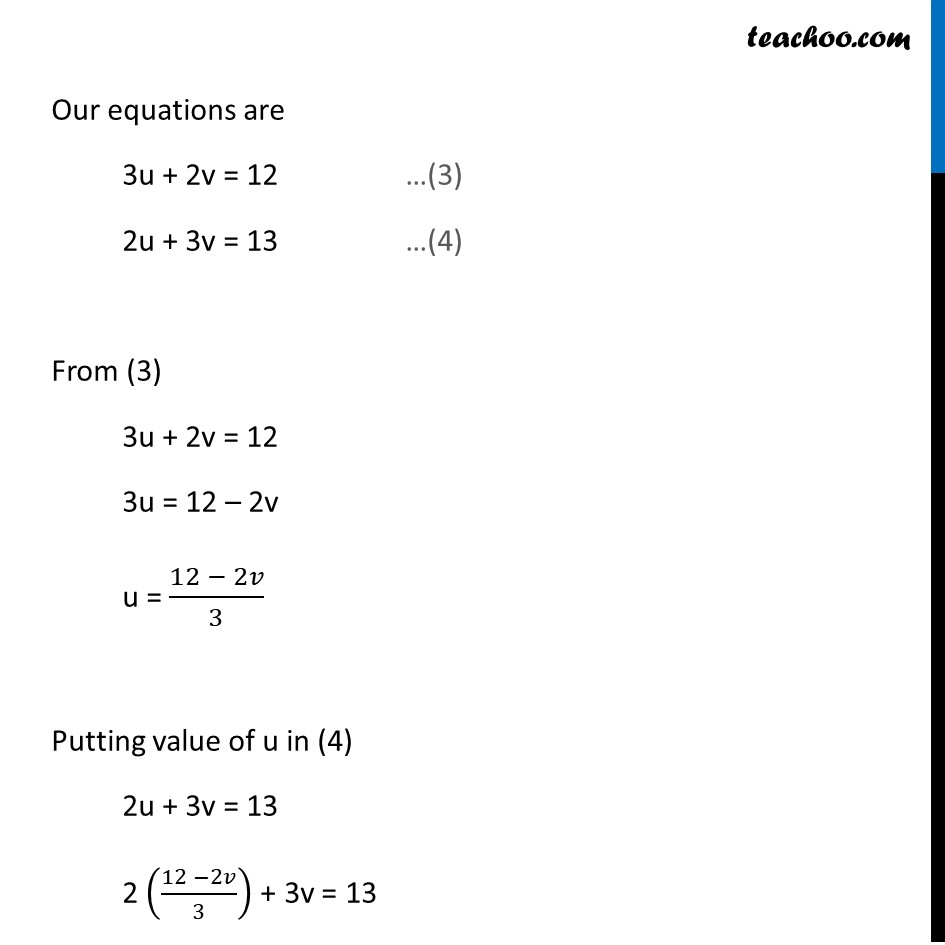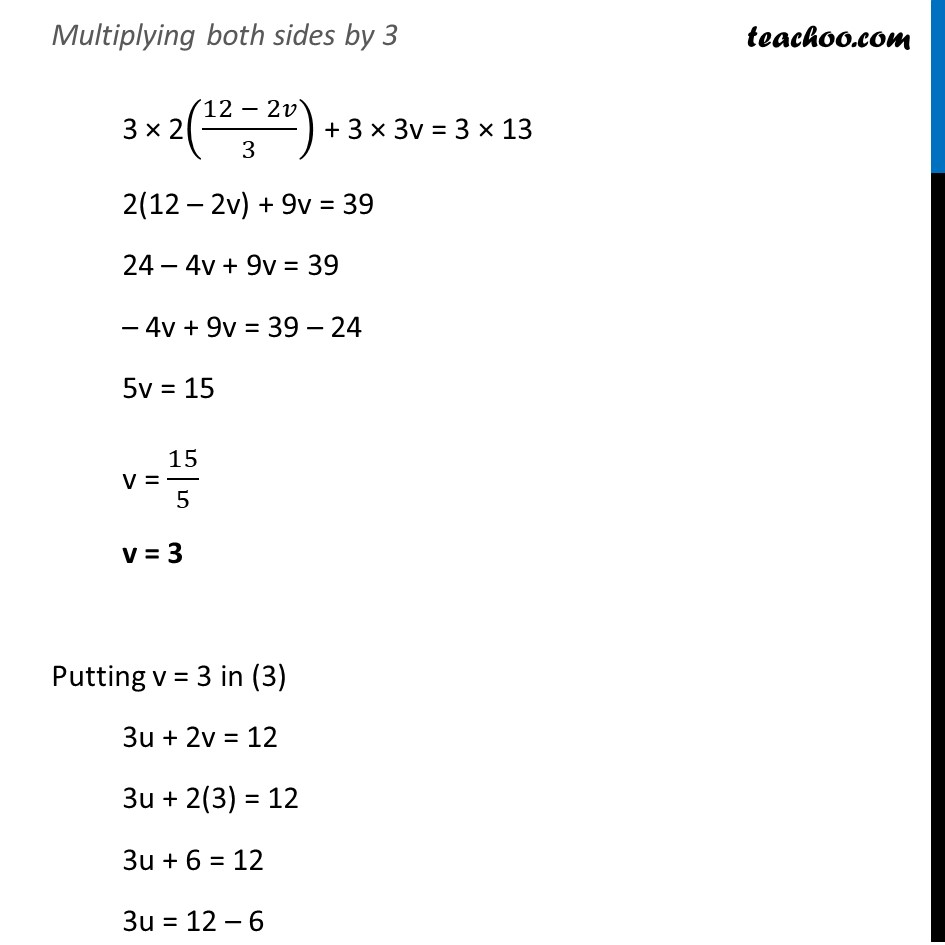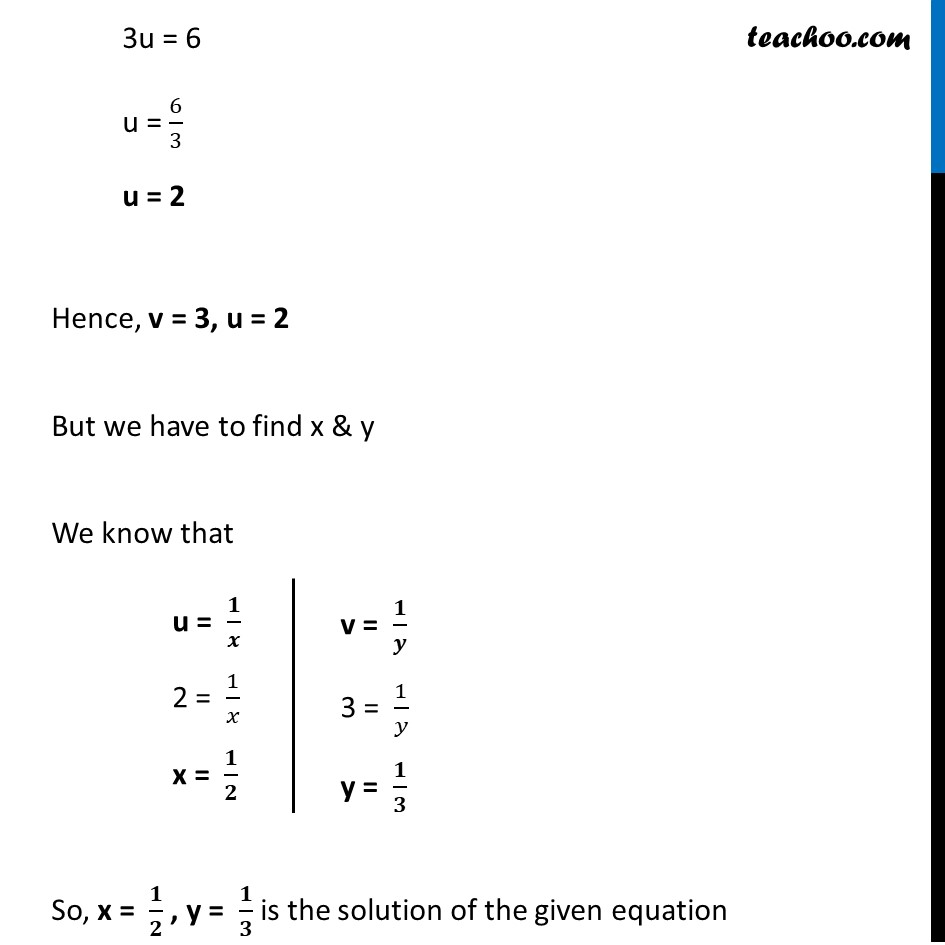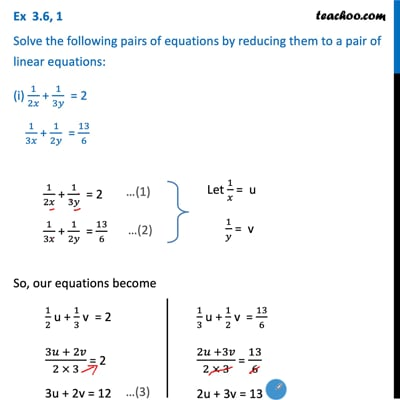This video is only available for Teachoo black users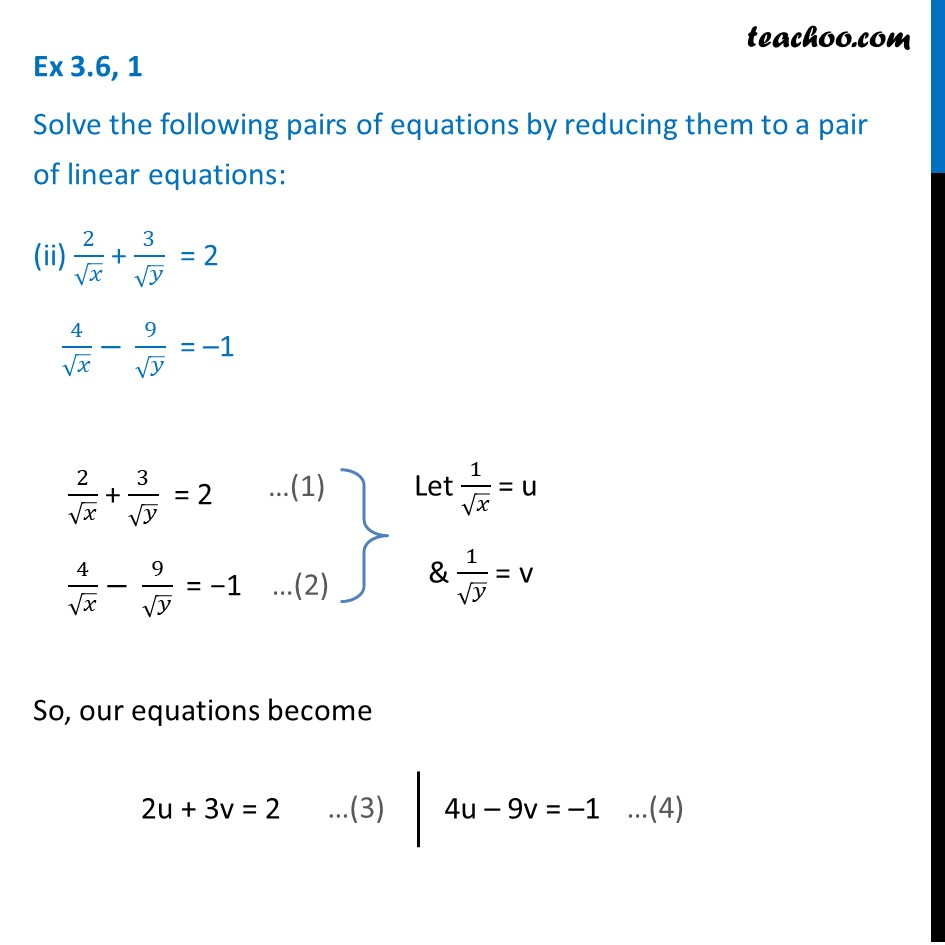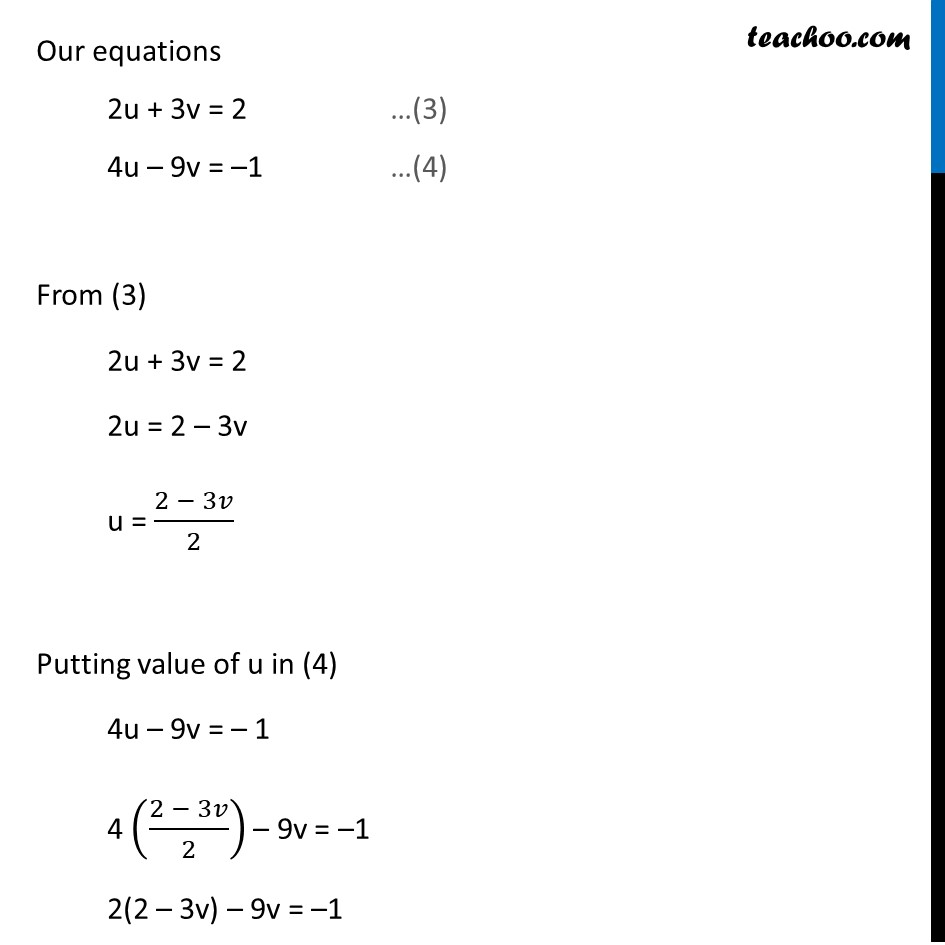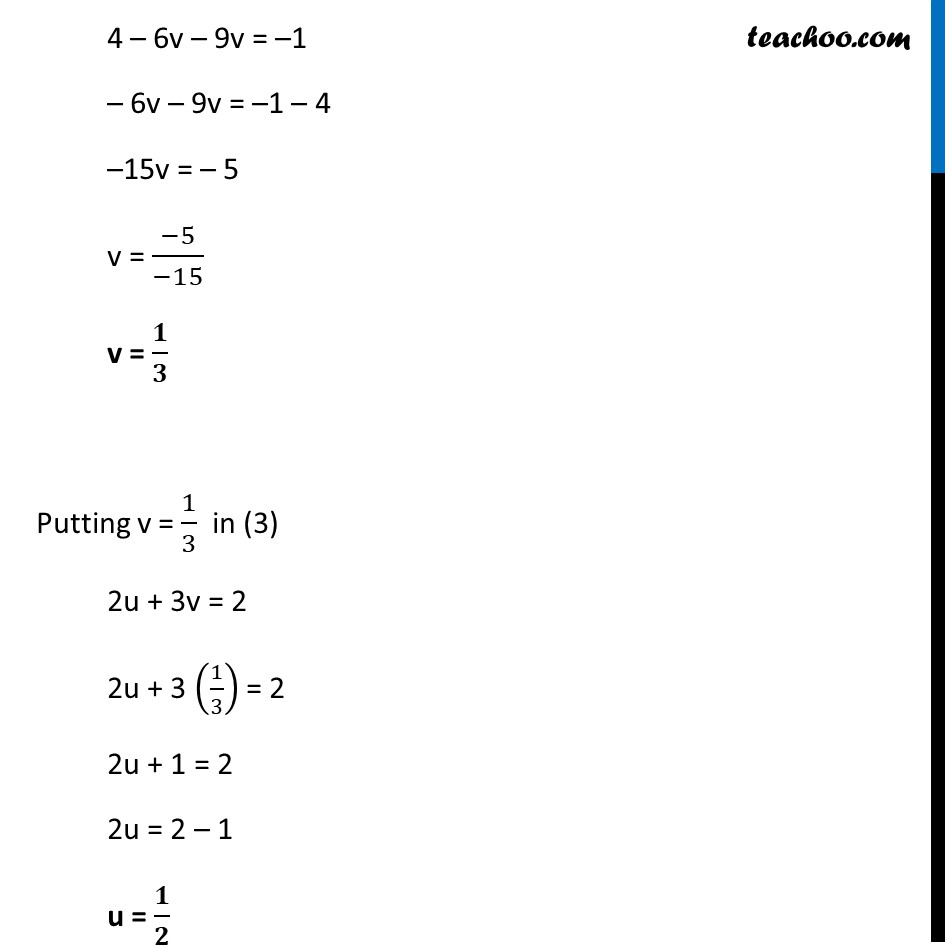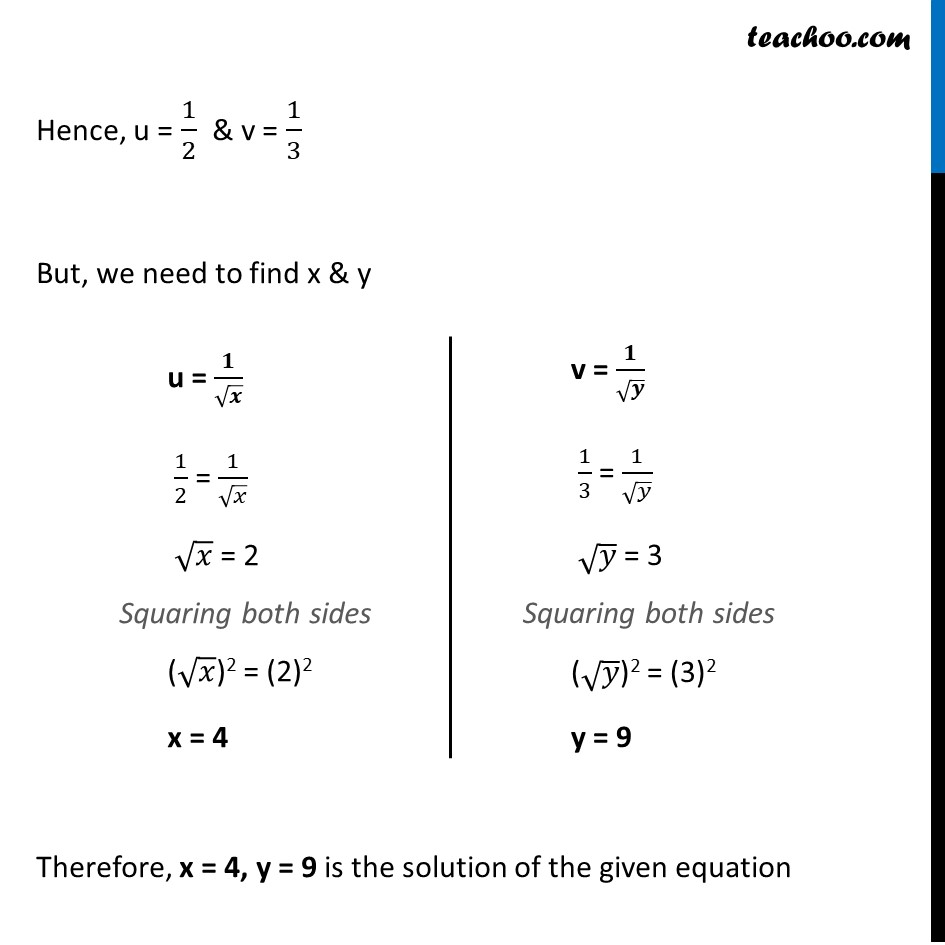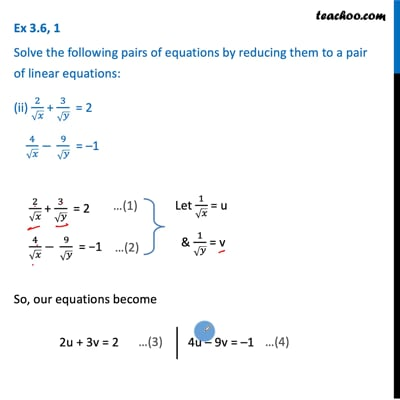This video is only available for Teachoo black users

Learn in your speed, with individual attention - Teachoo Maths 1-on-1 Class

### Transcript

Ex 3.6, 1 Solve the following pairs of equations by reducing them to a pair of linear equations: (i) 1/2𝑥 + 1/3𝑦 = 2 1/3𝑥 + 1/2𝑦 = 13/6 1/2𝑥 + 1/3𝑦 = 2 1/3𝑥 + 1/2𝑦 = 13/6 Let 1/𝑥 = u 1/𝑦 = v So, our equations become 1/2 u + 1/3 v = 2 (3𝑢 + 2𝑣)/(2 × 3) = 2 3u + 2v = 12 1/3 u + 1/2 v = 13/6 (2𝑢 +3𝑣)/(2 × 3) = 13/6 2u + 3v = 13 Our equations are 3u + 2v = 12 …(3) 2u + 3v = 13 …(4) From (3) 3u + 2v = 12 3u = 12 – 2v u = (12 − 2𝑣)/3 Putting value of u in (4) 2u + 3v = 13 2 ((12 −2𝑣)/3) + 3v = 13 Multiplying both sides by 3 3 × 2((12 − 2𝑣)/3) + 3 × 3v = 3 × 13 2(12 – 2v) + 9v = 39 24 – 4v + 9v = 39 – 4v + 9v = 39 – 24 5v = 15 v = 15/5 v = 3 Putting v = 3 in (3) 3u + 2v = 12 3u + 2(3) = 12 3u + 6 = 12 3u = 12 – 6 3u = 6 u = 6/3 u = 2 Hence, v = 3, u = 2 But we have to find x & y We know that u = 𝟏/𝒙 2 = 1/𝑥 x = 𝟏/𝟐 v = 𝟏/𝒚 3 = 1/𝑦 y = 𝟏/𝟑 So, x = 𝟏/𝟐 , y = 𝟏/𝟑 is the solution of the given equation Question 1 Solve the following pairs of equations by reducing them to a pair of linear equations: (ii) 2/√𝑥 + 3/√𝑦 = 2 4/√𝑥 − 9/√𝑦 = –1 2/√𝑥 + 3/√𝑦 = 2 4/√𝑥 − 9/√𝑦 = −1 So, our equations become 2u + 3v = 2 4u – 9v = –1 Our equations 2u + 3v = 2 …(3) 4u – 9v = –1 …(4) From (3) 2u + 3v = 2 2u = 2 – 3v u = (2 − 3𝑣)/2 Putting value of u in (4) 4u – 9v = – 1 4 ((2 − 3𝑣)/2) – 9v = –1 2(2 – 3v) – 9v = –1 4 – 6v – 9v = –1 – 6v – 9v = –1 – 4 –15v = – 5 v = (−5)/(−15) v = 𝟏/𝟑 Putting v = 1/3 in (3) 2u + 3v = 2 2u + 3 (1/3) = 2 2u + 1 = 2 2u = 2 – 1 u = 𝟏/𝟐 Hence, u = 1/2 & v = 1/3 But, we need to find x & y u = 𝟏/√𝒙 1/2 = 1/√𝑥 √𝑥 = 2 Squaring both sides (√𝑥)2 = (2)2 x = 4 v = 𝟏/√𝒚 1/3 = 1/√𝑦 √𝑦 = 3 Squaring both sides (√𝑦)2 = (3)2 y = 9 Therefore, x = 4, y = 9 is the solution of the given equation